Courses

# Test: Simple Stress & Strain Level - 1

## 20 Questions MCQ Test Strength of Materials (SOM) | Test: Simple Stress & Strain Level - 1

Description
This mock test of Test: Simple Stress & Strain Level - 1 for Mechanical Engineering helps you for every Mechanical Engineering entrance exam. This contains 20 Multiple Choice Questions for Mechanical Engineering Test: Simple Stress & Strain Level - 1 (mcq) to study with solutions a complete question bank. The solved questions answers in this Test: Simple Stress & Strain Level - 1 quiz give you a good mix of easy questions and tough questions. Mechanical Engineering students definitely take this Test: Simple Stress & Strain Level - 1 exercise for a better result in the exam. You can find other Test: Simple Stress & Strain Level - 1 extra questions, long questions & short questions for Mechanical Engineering on EduRev as well by searching above.
QUESTION: 1

### If a rod expands freely due to heating, it will develop

Solution: If a material expands or contract freely due to heating or cooling, then no stress will develop in the material. But if this expansion and contraction is prevented then internal and because of these internal resisting forces thermal stress develops in the material.

QUESTION: 2

### The ability of a material to deform without breaking is called

Solution:

The quality of being easily shaped or moulded is called Plasticity. So Plasticity is the ability of the material to deform without breaking.

QUESTION: 3

### When mild steel is subjected to a tensile load, its fracture will conform to

Solution:
QUESTION: 4

What is the relationship between elastic constants E, G and K?

Solution: E = 3K(1 − 2μ)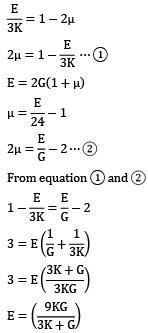From equation 1 and 2QUESTION: 5

Materials which show direction dependent properties are called

Solution: Homogeneous Material: Those materials which have the same properties at all the points.

Viscoelastic Materials:-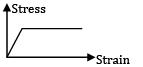Isotropic Materials: Those materials which have the same elastic properties in all the directions.

Anisotropic Materials: Those materials which show direction dependent properties are called anisotropic materials

QUESTION: 6

The number of elastic constants for a completely anisotropic elastic material is

Solution: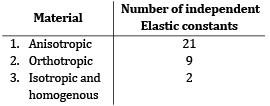QUESTION: 7

Match List-I (Elastic properties of an isotropic elastic material) with List-II (Nature of strain

produced) and select the correct answer using the codes given below the lists.

List-I

a. Young’s modulus

b. Modulus of rigidity

c. Bulk modulus

d. Poisson’s

List-II

1. Shear strain

2. Normal strain

3. Transverse strain

4. Volumetric strain

Codes: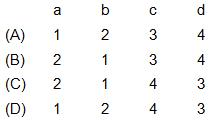Solution: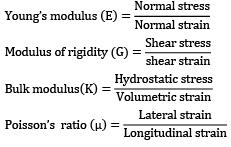QUESTION: 8

An isotropic elastic material is characterized by

Solution: The independent elastic constant will be any two of E, G and μ.

QUESTION: 9

Shape of true stress-strain curve for a material depends on

Solution:
QUESTION: 10

Which one of the following expresses the total elongation of a bar of length L with a constant cross-section of A and modulus of elasticity E hanging vertically and subject to its own weight W?

Solution: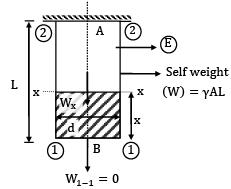Let γ is the weight density.

Wx−x = γAx

at x = 0 ⇒ W1−1 = 0

atx = L ⇒ W2−2 = γAL

Consider a strip at a distance x from section (1) − (1)of dx length

Elongation of the strip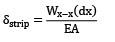Total elongation of bar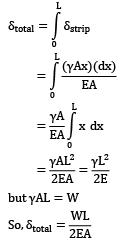QUESTION: 11

Which one of the following is correct in respect of Poisson’s ratio μ limits for an isotropic elastic solid?

Solution: For isotropic elastic solids, μ values can lie between −1 to 0.5 in order to satisfy positive values of E, G and K.

QUESTION: 12

A bar of copper and steel form a composite system which is heated through a temperature of 40℃. The stress induced in the copper bar is (αcopper > αsteel ).

Solution: As thermal coefficient of expansion (α) is more for copper in comparison to steel. So during heating, the tendency of copper is to elongate more, which will be restricted by steel. So copper will experience compressive stress while steel will experience tensile stress.

QUESTION: 13

The stress-strain curve of an ideal elastic strain hardening material will be as

Solution:
QUESTION: 14

A material which undergoes no deformation till its yield point is reached and then it flow at a constant stress is

Solution:
QUESTION: 15

In a simple tension test, Hooke’s law is valid upto the

Solution:

Hooke's law is valid upto the limit of proportionality .because it follows the straight-line equation upto the limit of proportionality. after the limit of proportionality, the elastic limit comes. between the limit of proportionality and elastic limit, the line follows the curve line. normally proportional limit and elastic limit very close to each other that's why we consider elastic limit instead of proportional limit for easy calculation.

QUESTION: 16

E, G, K and μ represent the elastic modulus, shear modulus, bulk modulus and poisson’s ratio respectively of a linearly elastic, isotropic and homogeneous material. To express the stress-strain relations completely for this material, at least

Solution: E = 3k (1 − 2μ) E = 2G (1 + μ) There are two equations and four unknown, so to express the stress-strain relations completely, at least any two of the four must be known. Remaining two can be found out by the equations.

QUESTION: 17

The Poission’s ratio of a material which has young’s modulus of 120 GPa and shear modulus of 50 GPa, is

Solution: E = 2G (1 + μ)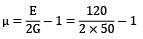μ = 0.2

QUESTION: 18

A block 100 mm x 100 mm base and 10 mm height. When we apply a tangential force 10 kN to the upper face, it is displaced 1 mm relative to lower face. Then the shear strain in the element is

Solution: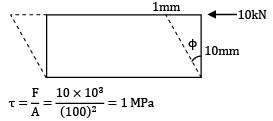tan ϕ = 1 / 10 = 0.1 radian

Question_Type: 5

QUESTION: 19

A block 100 mm 100 mm base and 10 mm height. When we apply a tangential force 10 kN to the upper edge it is displaced 1 mm relative to lower face.Then the direct shear stress in the element is:

Solution: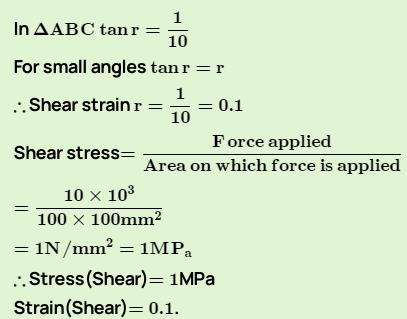QUESTION: 20

A 10 mm diameter of mild steel of elastic modulus 200 x 109Pa is subjected to a tensile load of 50000N, taking it just beyond its yield point. The elastic recovery of strain that would occur up on removal of tensile load will be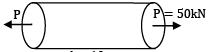d = 10mm

E = 200GPa

Tensile stress in the bar,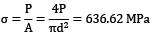Tensile strain will be elastic recovery because bar is loaded up to point of yield point,

∈=σ / E = 3.18 x 10−3